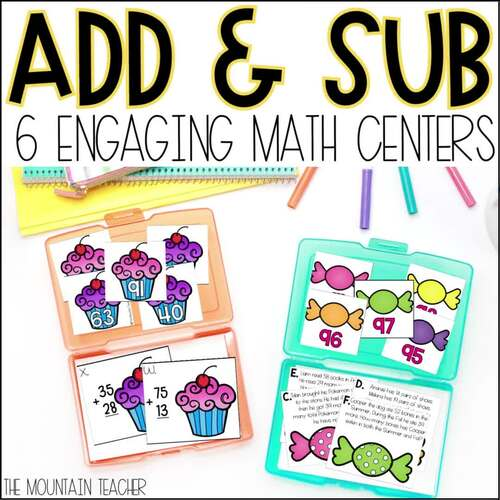# 2 Digit Addition and Subtraction with Regrouping Activities with Worksheets

Rated 5 out of 5, based on 21 reviews
21 Ratings;
2nd - 3rd
Subjects
Resource Type
Standards
Formats Included
• Zip
Pages
60+
\$9.99
List Price:
\$14.00
You Save:
\$4.01
Bundle
\$9.99
List Price:
\$14.00
You Save:
\$4.01
Bundle
Report this resource to TPT

#### Products in this Bundle (5)

##### Also included in
1. Looking for the BEST 2nd grade math centers for your classroom? These 42 hands on math activities are PERFECT for your classroom! Cover every standard including: place value, 2 digit addition and subtraction with regrouping, geometry, fractions, measurement, telling time, US money and multiplication
Price \$69.60Original Price \$87.00Save \$17.40

### Description

Looking for the BEST 2 digit addition and subtraction with regrouping games for your 2nd or 3rd graders? Don't sleep on these adding and subtracting 2 digit number activities and math centers! Students will practice adding and subtracting 2 digit numbers in 6 different ways including 2 addition with regrouping games, 2 subtraction with regrouping games and 2 word problem games.

What's Inside: 6 Two Digit Addition and Subtraction with Regrouping Centers

• Two 2 Digit Addition with Regrouping Matching Games
• Two 2 Digit Subtraction with Regrouping Matching Games
• One 2 Digit Addition with Regrouping Word Problem Game
• One 2 Digit Subtraction with Regrouping Word Problem Game

Standards Covered in the 2 Digit Addition and Subtraction with Regrouping Games:

• 2.OA.A.1 Use addition and subtraction within 100 to solve one- and two-step word problems involving situations of adding to, taking from, putting together, taking apart, and comparing, with unknowns in all positions, e.g., by using drawings and equations with a symbol for the unknown number to represent the problem.
• 2.OA.B.2 Fluently add and subtract within 20 using mental strategies.2 By end of Grade 2, know from memory all sums of two one-digit numbers.
• 2.NBT.B.5 Fluently add and subtract within 100 using strategies based on place value, properties of operations, and/or the relationship between addition and subtraction.
• 2.NBT.B.9 Explain why addition and subtraction strategies work, using place value and the properties of operations

• Small Groups
• Independent Work
• Earlier Finishers
• Centers
• Unit Review
• Test Prep
• Spiral Reviewing

& so much more!

Teaching addition and subtraction to 1000? Check out my resource for a unit plan and more resources here: Addition and Subtraction to 1000 Unit

If you like what you see, check out my second grade math center bundle as well: Second Grade Common Core Math Centers BUNDLE

Find Other Math Centers Below:

Total Pages
60+
Included
Teaching Duration
1 month
Report this resource to TPT
Reported resources will be reviewed by our team. Report this resource to let us know if this resource violates TPT’s content guidelines.

### Standards

to see state-specific standards (only available in the US).
Fluently add and subtract within 100 using strategies based on place value, properties of operations, and/or the relationship between addition and subtraction.
Explain why addition and subtraction strategies work, using place value and the properties of operations.
Use addition and subtraction within 100 to solve one- and two-step word problems involving situations of adding to, taking from, putting together, taking apart, and comparing, with unknowns in all positions, e.g., by using drawings and equations with a symbol for the unknown number to represent the problem.
Fluently add and subtract within 20 using mental strategies. By end of Grade 2, know from memory all sums of two one-digit numbers.